Question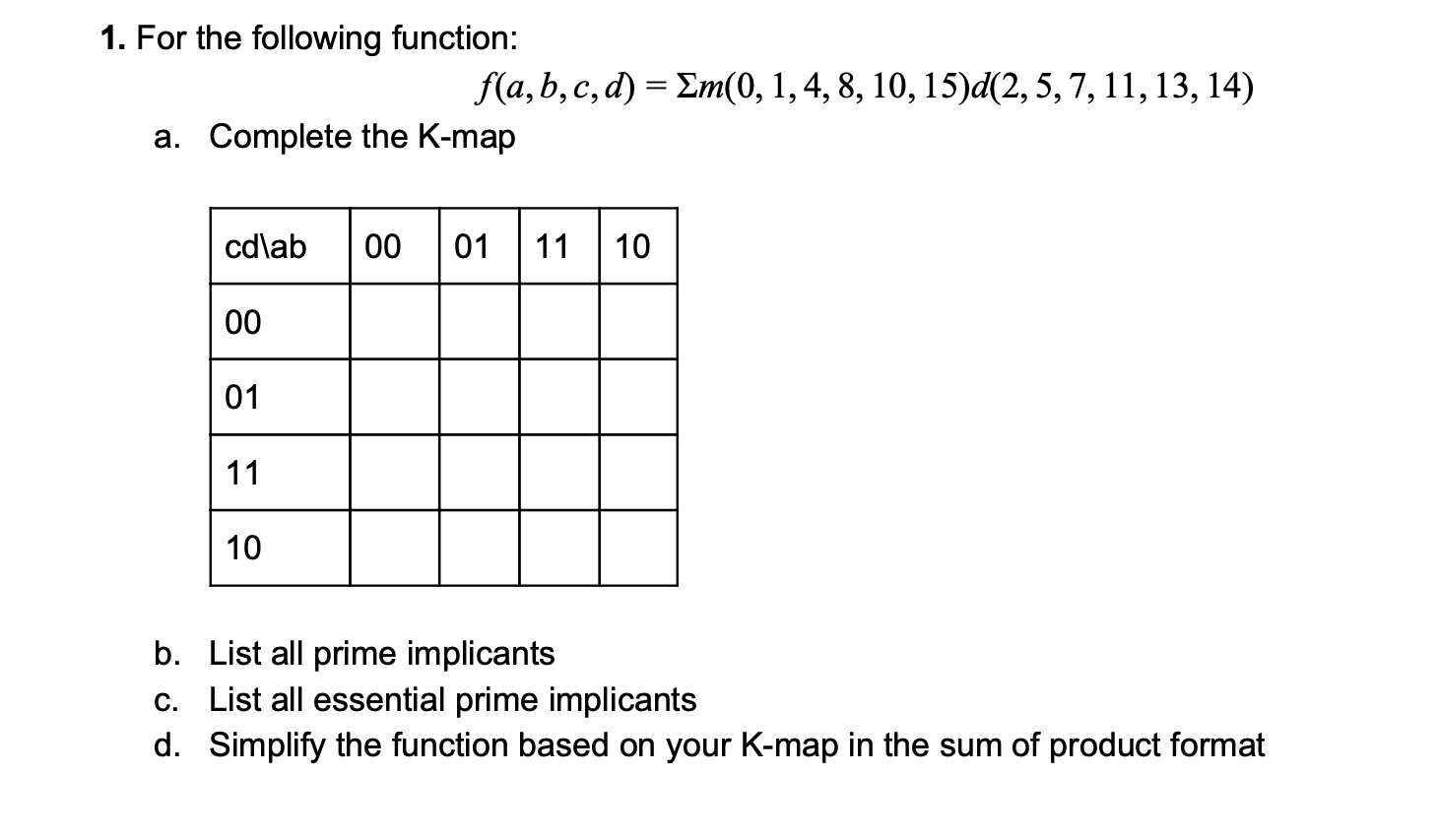Q>

a>

firstly i would like to tell you about the k map layout or index to put value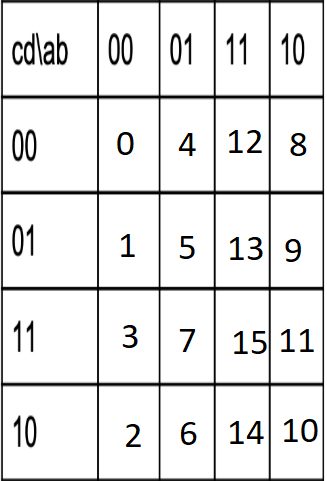after filling sop in k map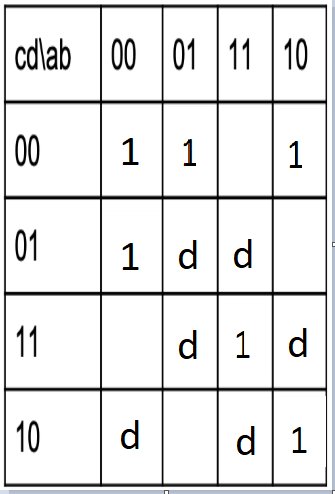b>list of all prime implication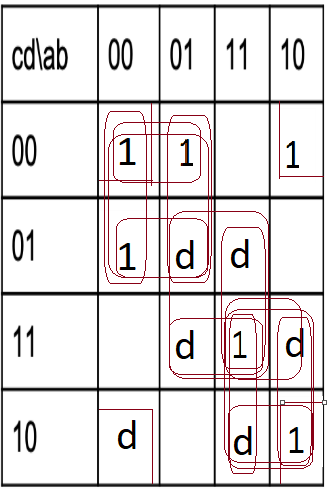14 prime implication

1>A'C'(0,1,4,5)

2>AC(10,11,14,15)

3>BCD(7,15)

4>A'C'D(1,5)

5>A'C'D'(0,4)

6>A'BC'(4,5)

7>A'B'C'(0,1)

8>ABD(13,15)

9>BD(5,7,13,15)

10>ACD'(10,14)

11>AB'C(10,11)

12>ABC(14,15)

13>ACD(11,15)

14>B'D'(0,2,8,10)

c>list of all essential prime implicants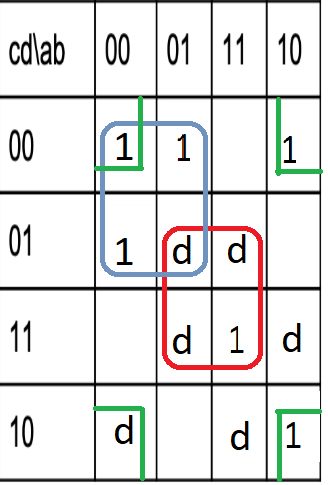1>A'C'(0,1,4,5)

2>BD(5,7,13,15)

3>B'D'(0,2,8,10)

d>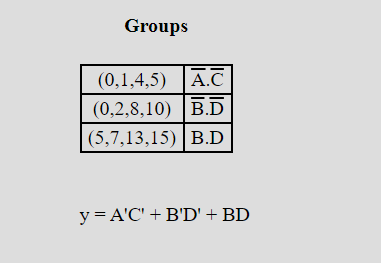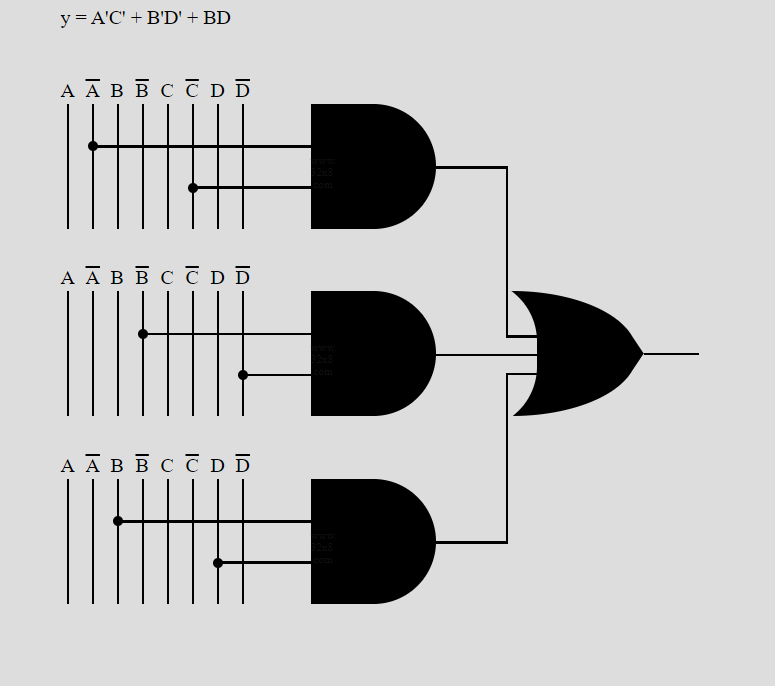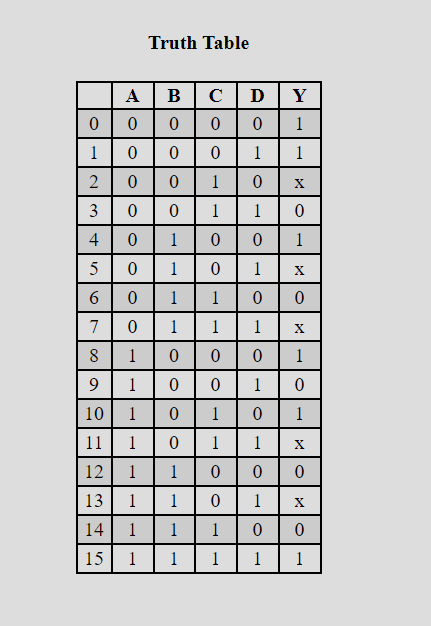Y=A'C'+BD+B'D'

PLEASE UPVOTE OR LIKE MY WORK .IF YOU HAVE ANY QUERY ASK IN THE COMMENT SECTION AS SOON AS POSSIBLE I REPLIED TO YOU.

PLEASE UPVOTE OR LIKE

#### Earn Coins

Coins can be redeemed for fabulous gifts.

Similar Homework Help Questions
• ### 2. Minimize the function F(a,b,c,d) = m(0,2,6,10,11,13,15) + d(1,4) (d=don't cares) using both the K- map...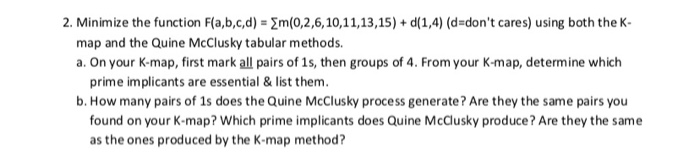2. Minimize the function F(a,b,c,d) = m(0,2,6,10,11,13,15) + d(1,4) (d=don't cares) using both the K- map and the Quine McClusky tabular methods. a. On your K-map, first mark all pairs of 1s, then groups of 4. From your K-map, determine which prime implicants are essential & list them. b. How many pairs of 1s does the Quine McClusky process generate? Are they the same pairs you found on your K-map? Which prime implicants does Quine McClusky produce? Are they the...

• ### 1. (10 point 1 effort points) Simplify the Boolean function F(A, B,C, D) - 11 (3,4,6,7,1...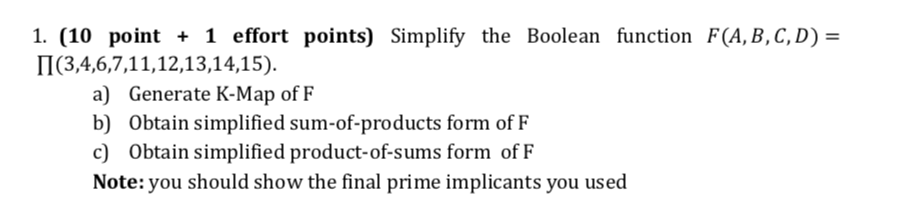1. (10 point 1 effort points) Simplify the Boolean function F(A, B,C, D) - 11 (3,4,6,7,1 1,12,13,14,15). a) Generate K-Map of F b) Obtain simplified sum-of-products form of F c) Obtain simplified product-of-sums form of F Note:you should show the final prime implicants you used

• ### uestion Completion Status QUESTION 1 What are the essential prime implicants for the logic function fa,b,c,d)...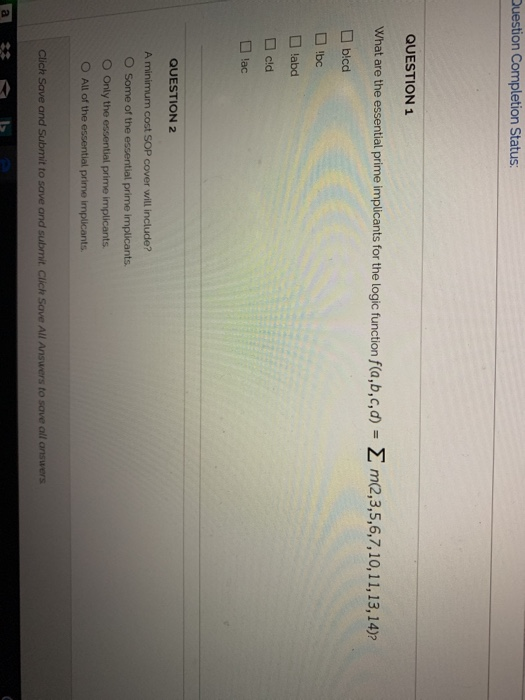uestion Completion Status QUESTION 1 What are the essential prime implicants for the logic function fa,b,c,d) □ blcd Σ m(2,3,5,6,7, 10, 11, 13, 14)? labd □cid lac QUESTION 2 A minimum cost SOP cover will include? O Some of the essential prime implicants O Only the essential prime implicants O All of the essential prime implicants Clich Save and Submit to save and submit Click Save All Answers to save all answers

• ### 8. A. For each of the following K-Maps (i) Identify minterms that cannot be covered by...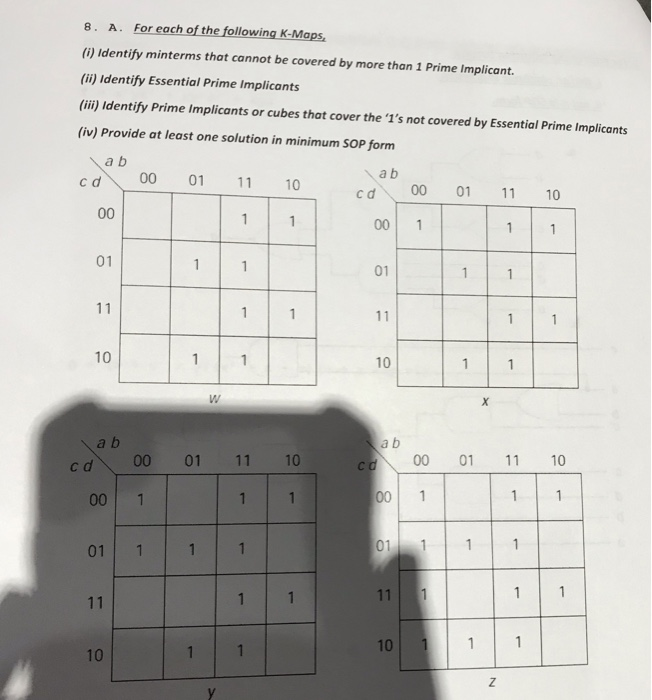8. A. For each of the following K-Maps (i) Identify minterms that cannot be covered by more than 1 Prime Implicant. (ii) Identify Essential Prime Implicants (ii) Identify Prime Implicants or cubes that cover the '1's not covered by Essential Prime Implicants (iv) Provide at least one solution in minimum SOP form a b a b c d 00 01 11 10 00 01 1 10 00 1 01 01 10 10 a b a b c d 00 01...

• ### (18 pts) Given the Boolean function F(A, B, C, D) = Σ (0, 1, 2, 3,...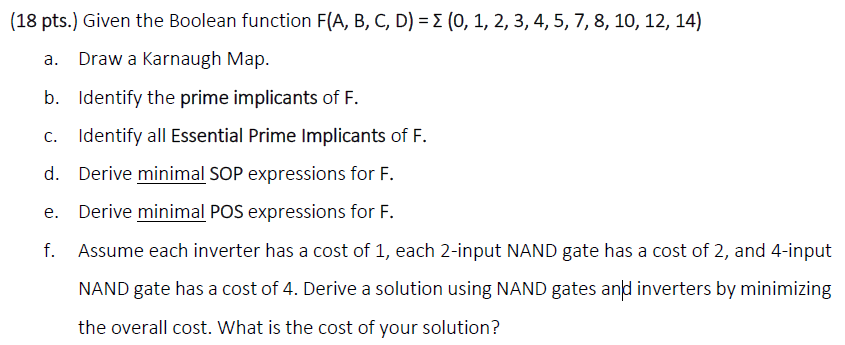(18 pts) Given the Boolean function F(A, B, C, D) = Σ (0, 1, 2, 3, 4, 5, 7, 8, 10, 12, 14) a. Draw a Karnaugh Map. b. Identify the prime implicants of F. c. Identify all Essential Prime Implicants of F. d. Derive minimal SOP expressions for F e. Derive minimal POS expressions for F. f. Assume each inverter has a cost of 1, each 2-input NAND gate has a cost of 2, and 4-input NAND gate has...

• ### Assume a function F(a,b,c) is 0 when abc-010 or abc=101, and is 1 when abc are...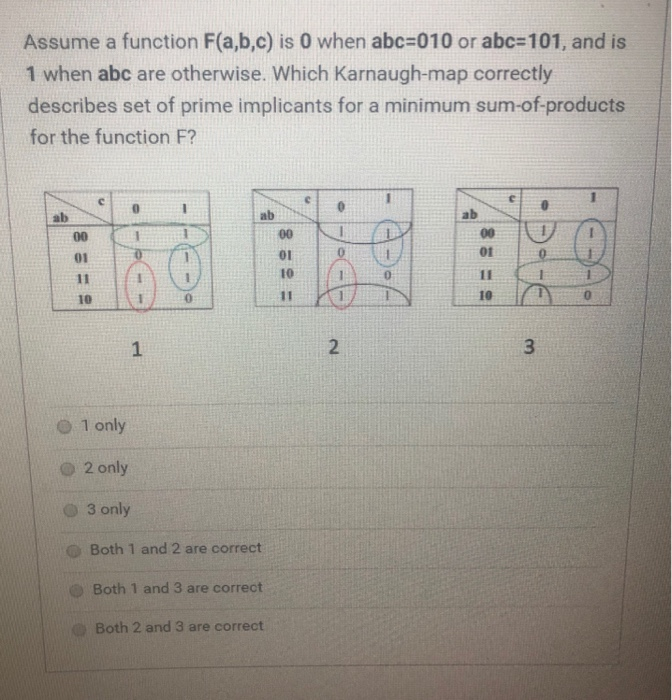Assume a function F(a,b,c) is 0 when abc-010 or abc=101, and is 1 when abc are otherwise. Which Karnaugh-map correctly describes set of prime implicants for a minimum sum-of-products for the function F? C ab ab ab 00 00 00 01 01 10 11 11 10 11 10 3 2 1 O 1 only 2 only 03 only Both 1 and 2 are correct Both 1 and 3 are correct Both 2 and 3 are correct

• ### Simplify the following K-map: F(A,B,C,D,E) = 2(0,1,2,3,8,10,13,15,16,17,18,19,24,26,29) A=0 00 01 11 A=1 DE BC0001 11 10...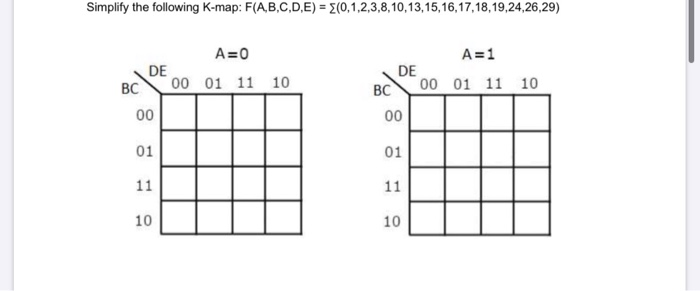Simplify the following K-map: F(A,B,C,D,E) = 2(0,1,2,3,8,10,13,15,16,17,18,19,24,26,29) A=0 00 01 11 A=1 DE BC0001 11 10 10

• ### Enter the following expression into a K-map: F(a,b,c,d) = Sum-of-minterms(1,3,4,5,7,8,12,15) Which of the following is not...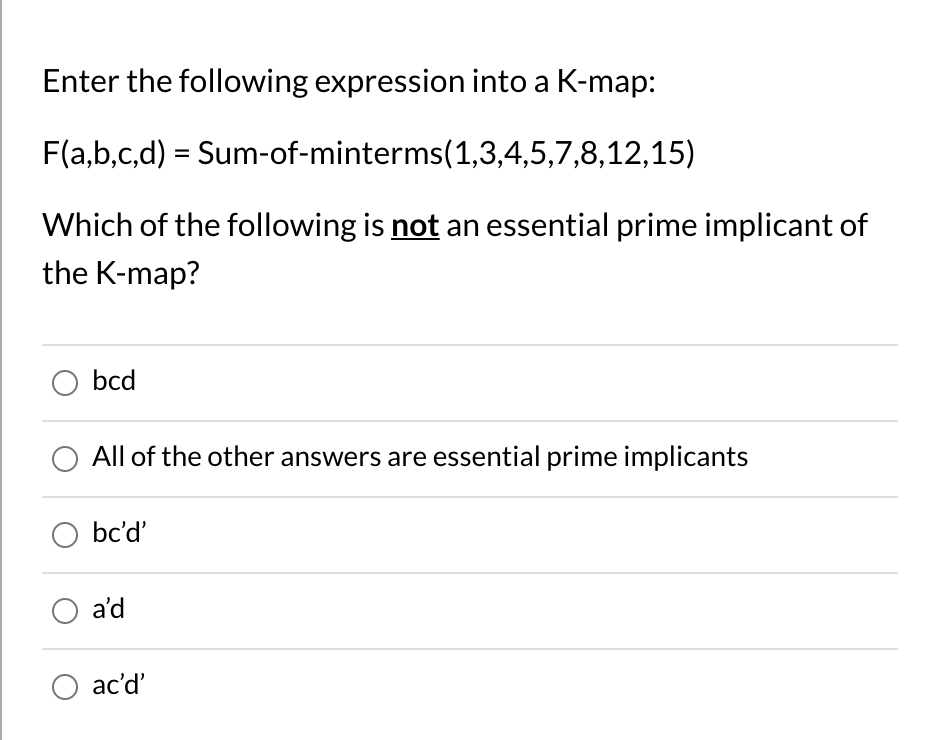Enter the following expression into a K-map: F(a,b,c,d) = Sum-of-minterms(1,3,4,5,7,8,12,15) Which of the following is not an essential prime implicant of the K-map? bcd All of the other answers are essential prime implicants bc'd' O a'd O ac'd'

• ### Please answer question 7. Question 3 is provided to answer question 7. Clearly write what F',...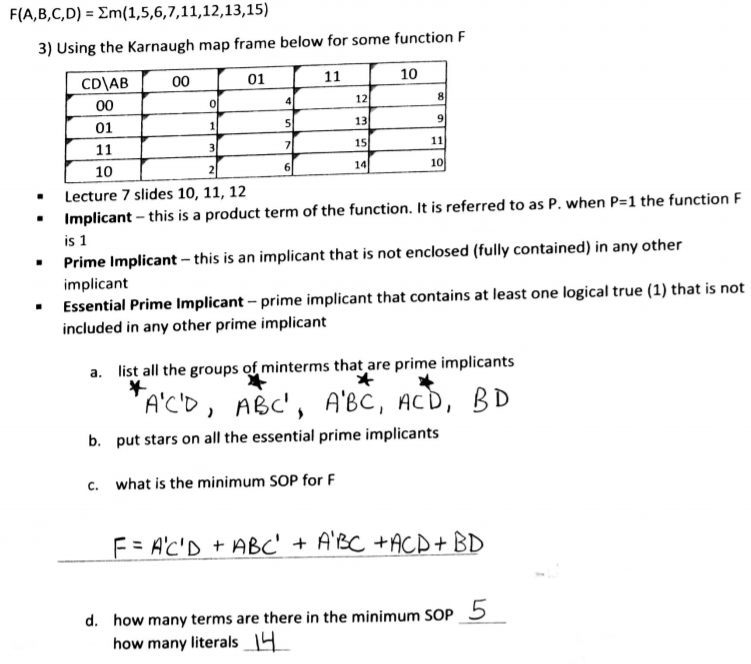Please answer question 7. Question 3 is provided to answer question 7. Clearly write what F', F, number of terms, and number of literals are. F(A,B,C,D) = Σm(1,5,6,7, 11, 12, 13,15) 3) Using the Karnaugh map frame below for some function F 01 10 CD\AB 12 0 13 9 01 14 10 Lecture 7 slides 10, 11,12 implicant-this is a product term of the function. It is referred to as P. when Ps] the function F is 1 · .Prime...

• ### AB 00 01 11 10 CD 00 0 0 4 1 12 1 8 1...

AB 00 01 11 10 CD 00 0 0 4 1 12 1 8 1 01 1 1 5 1   13 1 9 1   11 3 1 7 0 15 0 11 0 10 2 0 6 0 14 0 10 1 Simplify F(A, B, C, D) using the zeros of the k-map to get F`, then use De Morgan’s formula to get F in product of sums and select the one that matches it from the following; a-...